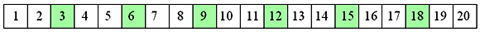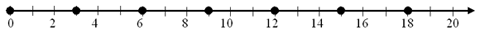Home > Patterns > Misunderstandings > Number lines > Number lines and repeating patterns

# Number lines and repeating patterns

The following activities can help students understand the number line better.

• Link the number line to skip counting.
• When counting repeating patterns, represent the numbers on a number track.
The equal spacing on the number track helps students to skip count (in this case, by threes).Counting by threes on a number track.

• Then move on to a number line (note the addition of zero at the beginning).Counting by threes on a number line.

• Gradually remove some of the labels, leaving numbers spaced by two, five or ten. In the following example, the numbers are spaced by two.Counting by threes on a different number line.

• Later, remove the intermediate marks as well.

• Plot timelines.
• Plot the Olympic years on a timeline marked at one-year intervals but numbered every five years.
• Plot recent significant events on a timeline marked and numbered at two-year intervals.
• Plot significant daily events (from waking to sleeping) on a 24-hour timeline.